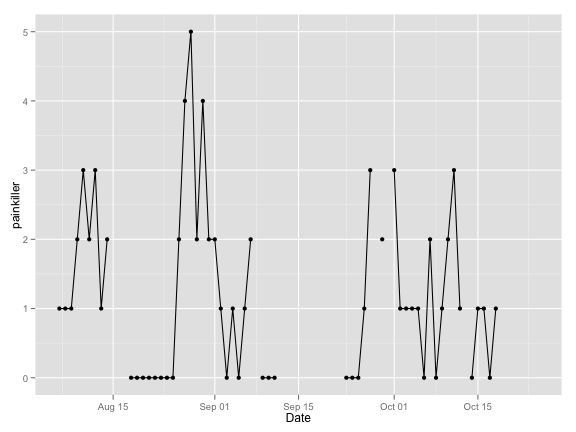# Ellie's Log

The results here are also shown on SlideShare with some annotation.

My daughter, ellie, was born in January. Since her birth, we have been keeping detailed records of her sleep, feeding and diapers. In honor of QS2012, I digitized our log and have put together some plots of the key variables that we measured.

changes made as a test.``````# setwd('~/Desktop/Dropbox/EllieLog/')
require(ggplot2)
``````
``````## Loading required package: ggplot2
``````
``````require(reshape2)
``````
``````## Loading required package: reshape2
``````
``````
# read in log data
Ellielog = read.csv("Ellielog2.csv")

# reformat dates
dates = seq(from = as.Date("2012-01-19"), to = as.Date("2012-10-18"), by = "day")
Ellielog\$Date = dates

# create age in days variate
Ellielog\$age = as.numeric(Ellielog\$Date - Ellielog\$Date)

# read in weight data
EllieWeight = read.csv("ellieWeight.csv")
names(EllieWeight) = "Date"
EllieWeight\$Date = as.Date(EllieWeight\$Date)

# merge weight data into log
Ellielog = merge(Ellielog, EllieWeight[, c(1, 4)], all.x = TRUE, all.y = TRUE)

# manual additions of weigh ins excluded from chart
Ellielog\$weight.lb = 7.5
Ellielog\$weight.lb = 7
Ellielog\$weight.lb = 6.75
Ellielog\$weight.lb = 6.9375
Ellielog\$weight.lb = 6.9375
Ellielog\$weight.lb = 7 + 5/16
Ellielog\$weight.lb = 7 + 15.5/16

# getting the 50th perctile for weight from CDC equations
cdcCoefs = c(3.23249, 0.764466, -0.0115107, -0.000912367)
cdc50 = function(coef, age.in.days) {
ageM = age.in.days/30.4368
weight.kg = coef + coef * ageM + coef * ageM^2 + coef * ageM^3
weight.lb = weight.kg * 2.2
return(weight.lb)
}
Ellielog\$percentile50 = cdc50(cdcCoefs, 1:dim(Ellielog))

# create the melted Ellielog
Ellielog.melt = melt(Ellielog, id.vars = "Date")
``````

## Weight

``````qplot(Date, value, data = subset(Ellielog.melt, variable %in% c("weight.lb",
"percentile50")), color = variable) + labs(y = "pounds", color = "") + scale_color_hue(h.start = 180)
``````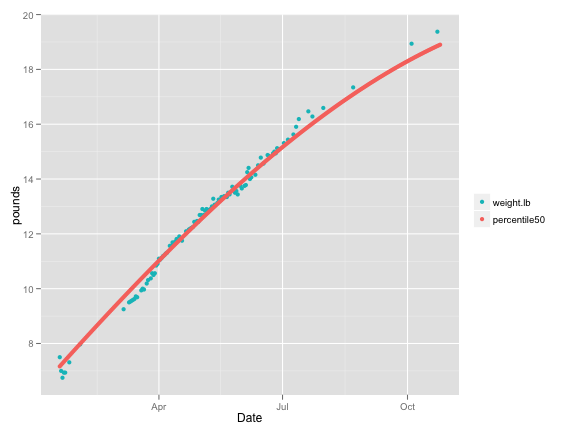``````qplot(Date, value, data = subset(Ellielog.melt, variable %in% c("weight.lb",
"percentile50") & Date < Ellielog\$Date), color = variable, main = "zoom in on first 100 days") +
annotate("segment", y = -Inf, yend = Inf, x = 15422, xend = 15422, color = "limegreen",
lty = 2) + labs(y = "pounds", color = "") + scale_color_hue(h.start = 180) +
annotate("text", x = 15432, y = 8.25, label = "start meds", color = "limegreen")
``````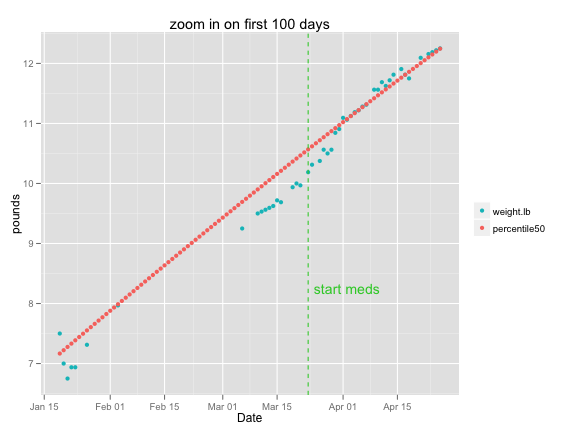## Sleep

``````qplot(Date, LSP, data = Ellielog, geom = c("point", "smooth"), main = "Longest Sleep Period (hours)")
``````
``````## geom_smooth: method="auto" and size of largest group is <1000, so using
## loess. Use 'method = x' to change the smoothing method.
``````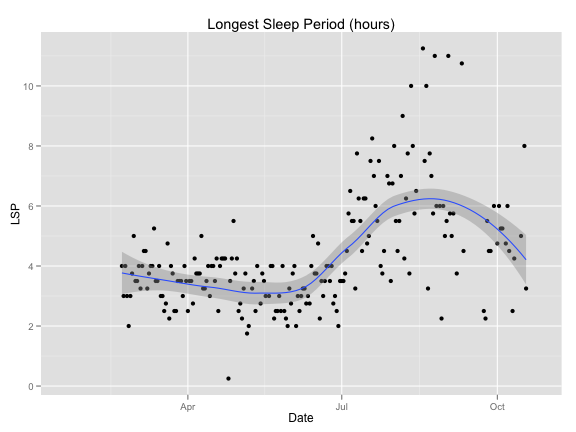``````qplot(Date, nightSleep, data = Ellielog, geom = c("point", "smooth"), main = "Night time sleep (hours)")
``````
``````## geom_smooth: method="auto" and size of largest group is <1000, so using
## loess. Use 'method = x' to change the smoothing method.
``````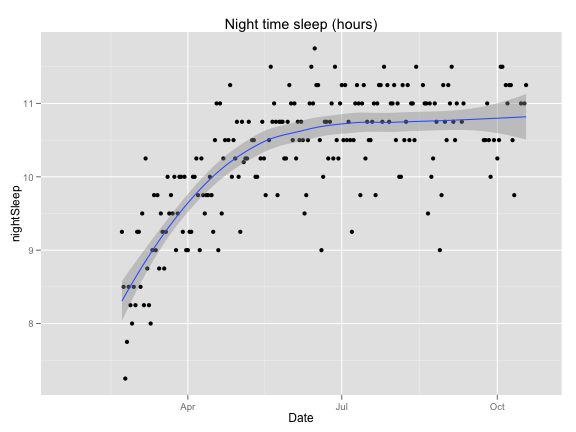``````qplot(Date, daySleep, data = Ellielog, geom = c("point", "smooth"), main = "Daytime sleep (hours)")
``````
``````## geom_smooth: method="auto" and size of largest group is <1000, so using
## loess. Use 'method = x' to change the smoothing method.
``````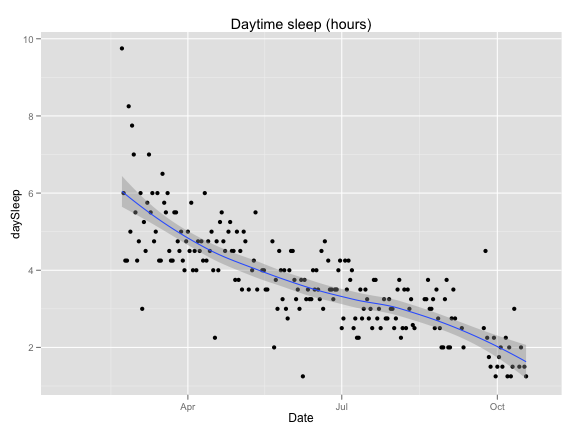``````qplot(Date, nightSleep + daySleep, data = Ellielog, geom = c("point", "smooth"),
main = "Total sleep (hours)")
``````
``````## geom_smooth: method="auto" and size of largest group is <1000, so using
## loess. Use 'method = x' to change the smoothing method.
``````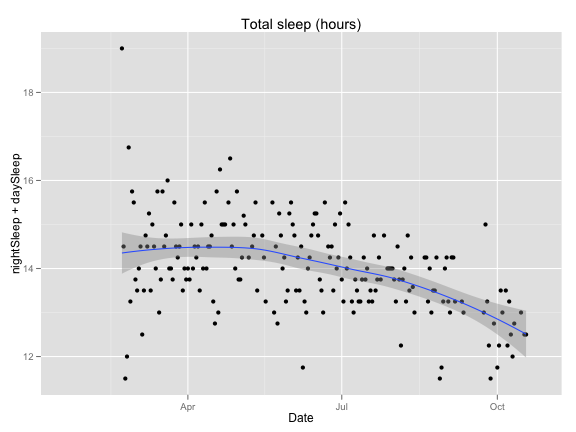``````qplot(Date, naps, data = Ellielog, geom = c("point", "smooth"), main = "Number of naps")
``````
``````## geom_smooth: method="auto" and size of largest group is <1000, so using
## loess. Use 'method = x' to change the smoothing method.
``````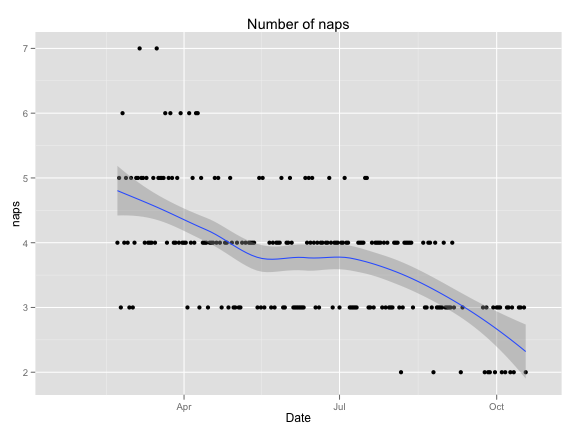``````qplot(Date, longestNap, data = Ellielog, geom = c("point", "smooth"), main = "Longest Nap (hours)")
``````
``````## geom_smooth: method="auto" and size of largest group is <1000, so using
## loess. Use 'method = x' to change the smoothing method.
``````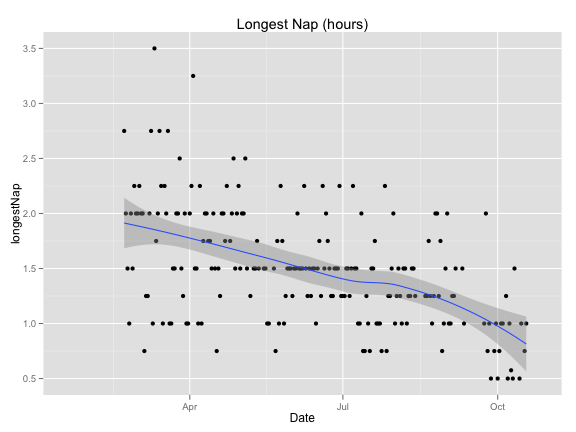``````qplot(nightSleep, daySleep, data = Ellielog, color = age) + annotate("segment",
x = 7, y = 7.5, xend = 12, yend = 2.5, lty = 2) + scale_color_gradient(low = "pink",
high = "red") + annotate("text", x = 7.5, y = 7.45, label = "14.5 hour line",
srt = 338) + labs(color = "age in days")
``````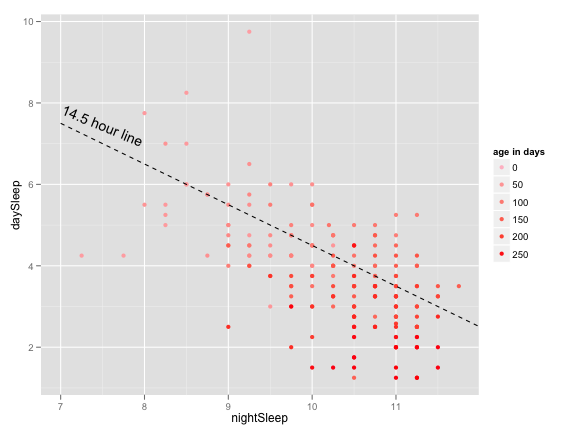``````qplot(Date, nightWakings, data = Ellielog, geom = c("point", "smooth"))
``````
``````## geom_smooth: method="auto" and size of largest group is <1000, so using
## loess. Use 'method = x' to change the smoothing method.
``````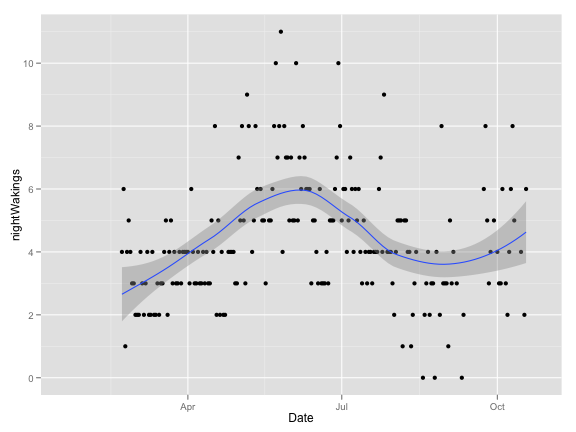## Diapers

``````qplot(Date, P, data = Ellielog, geom = c("point", "smooth"), main = "Number of poop diapers")
``````
``````## geom_smooth: method="auto" and size of largest group is <1000, so using
## loess. Use 'method = x' to change the smoothing method.
``````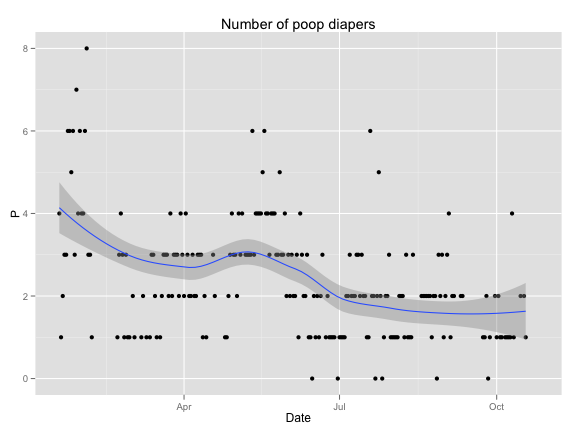## Feedings

``````qplot(Date, longestBF, data = Ellielog, geom = c("point", "smooth"), main = "Longest Breastfeeding (minutes)")
``````
``````## geom_smooth: method="auto" and size of largest group is <1000, so using
## loess. Use 'method = x' to change the smoothing method.
``````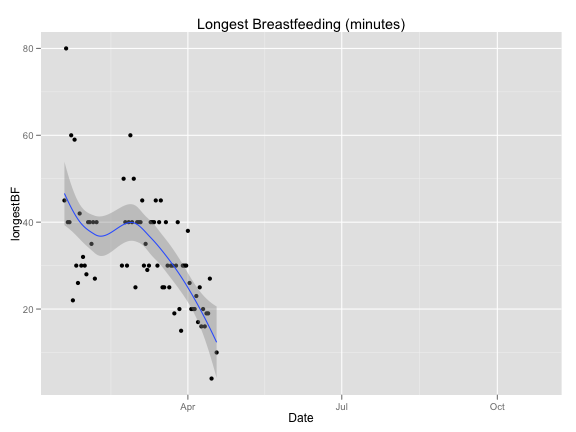``````Ellielog\$longestBF[-c(1:88)] = 10
qplot(Date, feedings, data = Ellielog, geom = c("point", "smooth"), main = "Number of feedings",
size = longestBF)
``````
``````## geom_smooth: method="auto" and size of largest group is <1000, so using
## loess. Use 'method = x' to change the smoothing method.
``````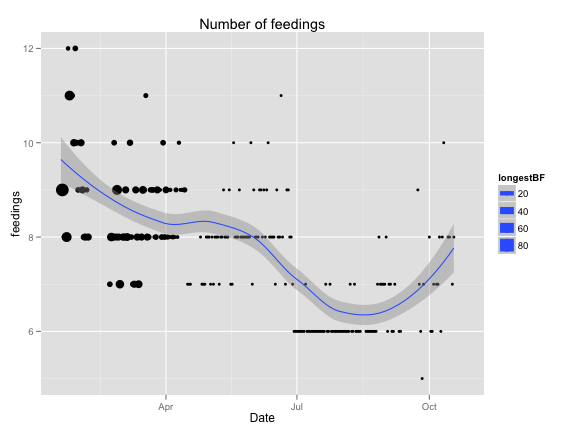``````qplot(Date, bottles, data = Ellielog, geom = c("point", "smooth"), main = "Number of bottle feedings")
``````
``````## geom_smooth: method="auto" and size of largest group is <1000, so using
## loess. Use 'method = x' to change the smoothing method.
``````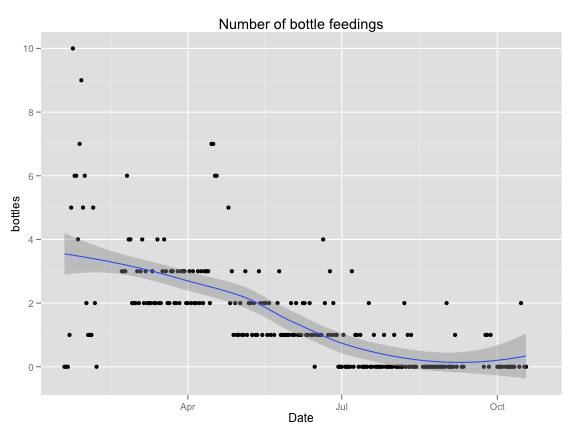``````qplot(Date, longestBF, data = Ellielog, geom = c("point", "smooth"), main = "Longest Breastfeeding (minutes)")
``````
``````## geom_smooth: method="auto" and size of largest group is <1000, so using
## loess. Use 'method = x' to change the smoothing method.
``````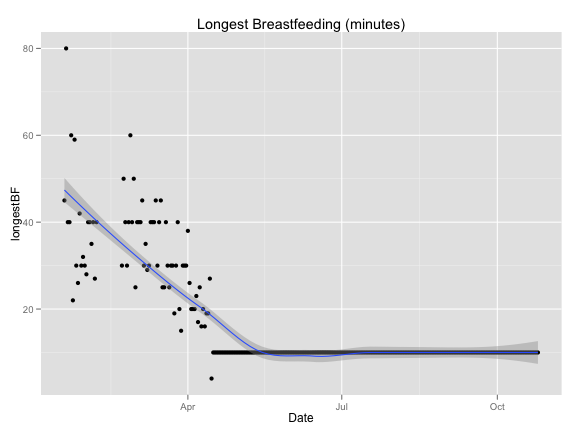``````qplot(Date, solids, data = Ellielog, geom = c("point", "smooth"), main = "Number of solids feedings")
``````
``````## geom_smooth: method="auto" and size of largest group is <1000, so using
## loess. Use 'method = x' to change the smoothing method.
``````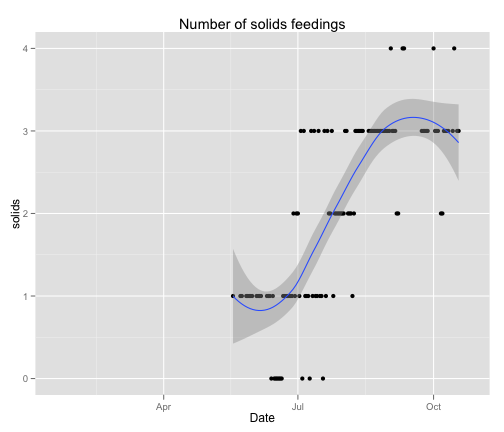## Teething

``````qplot(Date, painkiller, data = Ellielog[-c(1:200), ], geom = c("point", "line"))
``````